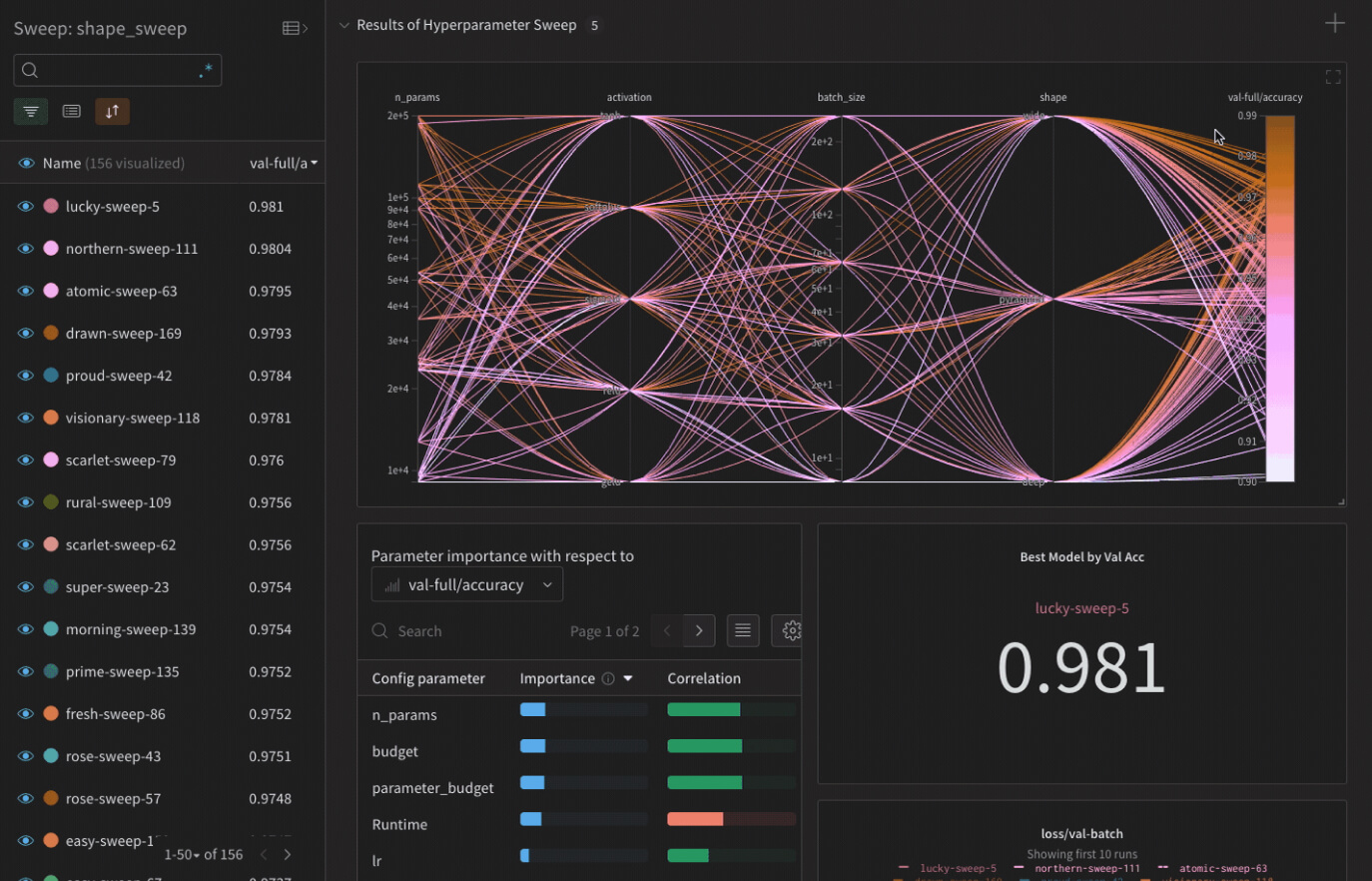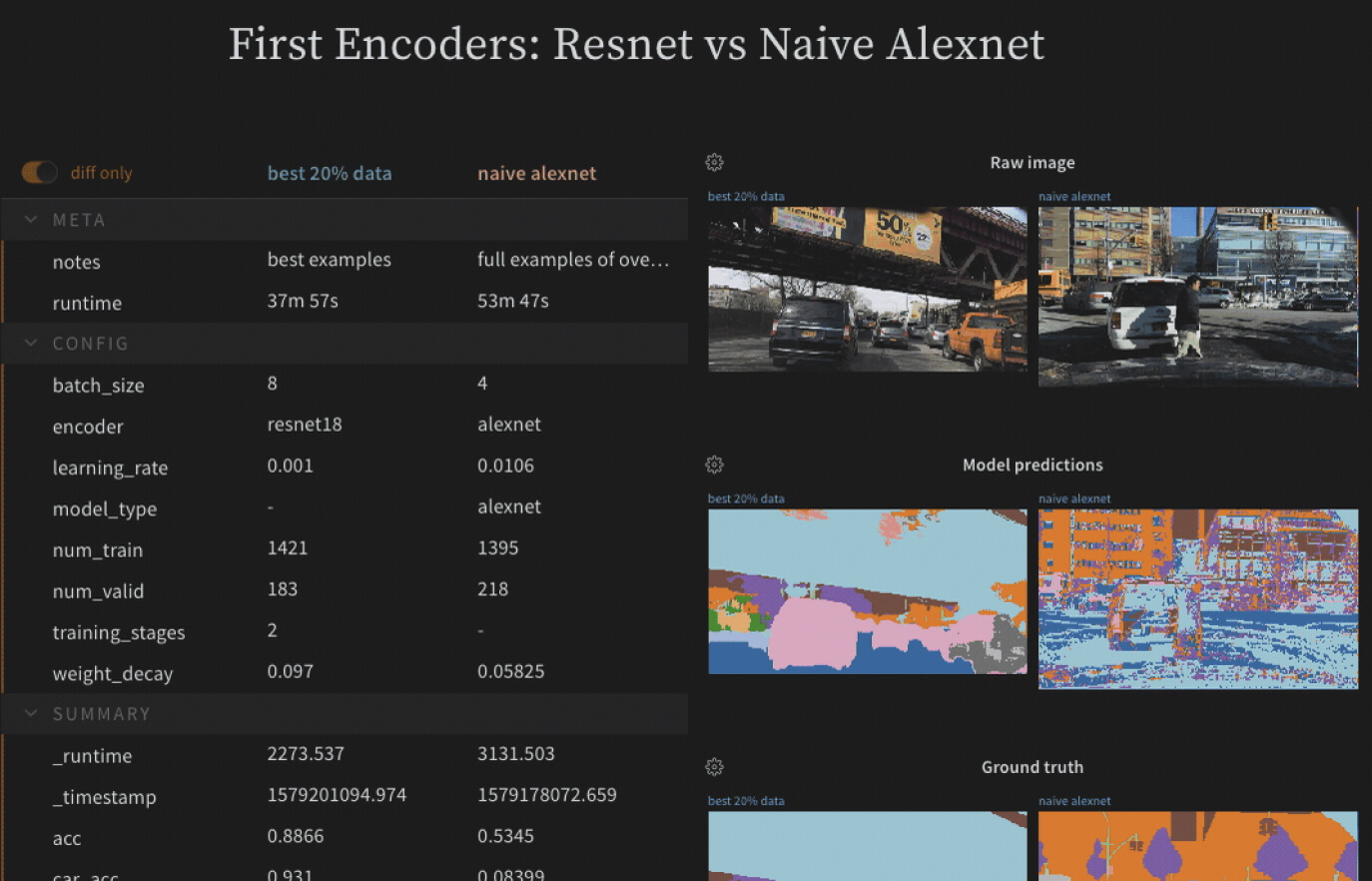01

## Integrate quickly

Track, compare, and visualize ML experiments with 5 lines of code. Free for academic and open source projects.

# Flexible integration for any Python script
import wandb
# 1. Start a W&B run
wandb.init(project='gpt4')
config = wandb.config
config.learning_rate = 0.01
# 2. Save model inputs and hyperparameters
# Model training here
# 3. Log metrics over time to visualize performance
wandb.log({"loss": loss})
import wandb
# 1. Start a W&B run
wandb.init(project='gpt3')
# 2. Save model inputs and hyperparameters
config = wandb.config
config.learning_rate = 0.01
# Model training here
# 3. Log metrics over time to visualize performance
with tf.Session() as sess:
# ...
wandb.tensorflow.log(tf.summary.merge_all())
import wandb
# 1. Start a new run
wandb.init(project="gpt-3")
# 2. Save model inputs and hyperparameters
config = wandb.config
config.learning_rate = 0.01
# 3. Log gradients and model parameters
wandb.watch(model)
for batch_idx, (data, target) in
if batch_idx % args.log_interval == 0:
# 4. Log metrics to visualize performance
wandb.log({"loss": loss})
import wandb
from wandb.keras import WandbCallback
# 1. Start a new run
wandb.init(project="gpt-3")
# 2. Save model inputs and hyperparameters
config = wandb.config
config.learning_rate = 0.01
... Define a model
# 3. Log layer dimensions and metrics over time
model.fit(X_train, y_train, validation_data=(X_test, y_test),
callbacks=[WandbCallback()])
import wandb
wandb.init(project="visualize-sklearn")
# Model training here
# Log classifier visualizations
wandb.sklearn.plot_classifier(clf, X_train, X_test, y_train, y_test, y_pred, y_probas, labels, model_name='SVC', feature_names=None)
# Log regression visualizations
wandb.sklearn.plot_regressor(reg, X_train, X_test, y_train, y_test,  model_name='Ridge')
# Log clustering visualizations
wandb.sklearn.plot_clusterer(kmeans, X_train, cluster_labels, labels=None, model_name='KMeans')
# 1. Import wandb and login
import wandb
# 2. Define which wandb project to log to and name your run
wandb.init(project="gpt-3", run_name='gpt-3-base-high-lr')
# 3. Add wandb in your Hugging Face `TrainingArguments`
args = TrainingArguments(... , report_to='wandb')
# 4. W&B logging will begin automatically when your start training your Trainer
trainer = Trainer(... , args=args)
trainer.train()
import wandb
# 1. Start a new run
wandb.init(project="visualize-models", name="xgboost")
# 2. Add the callback
bst = xgboost.train(param, xg_train, num_round, watchlist, callbacks=[wandb.xgboost.wandb_callback()])
# Get predictions
pred = bst.predict(xg_test)02

## Visualize seamlessly

Add W&B's lightweight integration to your existing ML code and quickly get live metrics, terminal logs, and system stats streamed to the centralized dashboard.

03

## Collaborate in real time

Explain how your model works, show graphs of how model versions improved, discuss bugs, and demonstrate progress towards milestones.